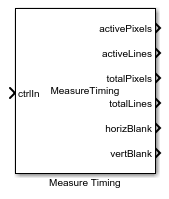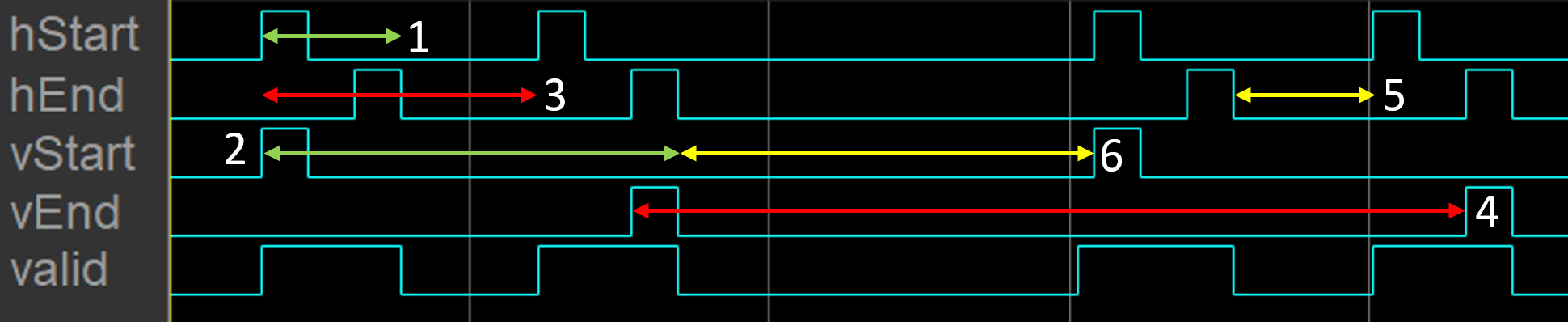Main Content

# Measure Timing

Measure timing of pixel control bus input

• Library:
• Vision HDL Toolbox / Utilities

•## Description

The Measure Timing block measures the timing parameters of a video stream. This block is for use in Simulink® simulation only and does not support HDL code generation.

The Vision HDL Toolbox™ streaming pixel protocol implements the timing of a video system, including inactive intervals between frames. These inactive intervals are called blanking intervals. Many Vision HDL Toolbox blocks require minimum blanking intervals. For more information, see Configure Blanking Intervals. You can use the timing parameter measurements from this block to check that your video stream meets these requirements. If you manipulate the control signals of your video stream, you can use this block to verify the resulting signals.

To determine the parameters of each frame, the block measures the time steps between the control signals on the bus.• `1` — Active pixels per line

• `2` — Active lines per frame (count `hStart` pulses)

• `3` — Total pixels per line

• `4` — Total lines per frame (cycles divided by total pixels per line)

• `5` — Horizontal blanking

• `6` — Vertical blanking (cycles, minus horizontal blanking, divided by total pixels per line)

For details on the pixel control bus and the dimensions of a video frame, see Streaming Pixel Interface.

Note

Measurements from the first simulated frame are incorrect because some parameters require measurements between frames. Simulate at least two frames before using the results.

## Ports

### Input

expand all

The `pixelcontrol` bus contains five signals. The signals describe the validity of the pixel and its location in the frame. For more information, see Pixel Control Bus.

Data Types: `bus`

### Output

expand all

Number of pixels in each line of the active video frame, returned as a scalar. This value is measured by counting valid pixels between `hStart` and `hEnd`. See marker `1` in the diagram.

Data Types: `double`

Number of lines in the active video frame, returned as a scalar. This value is measured by counting the `hStart` pulses between `vStart` and `vEnd`. See marker `2` in the diagram.

Data Types: `double`

Number of pixels in each line, including the horizontal blanking interval, returned as a scalar. This value is measured by counting the number of valid pixels between two `hStart` pulses. See marker `3` in the diagram.

Data Types: `double`

Number of lines in the frame, including the vertical blanking interval, returned as a scalar. This value is measured by counting the number of valid pixels between two `vStart` pulses, divided by `totalPixels`. See marker `4` in the diagram.

Data Types: `double`

Number of inactive pixels between lines of a frame, returned as a scalar. This value is measured by counting invalid cycles between `hEnd` and the next `hStart`. See marker `5` in the diagram.

Data Types: `double`

Number of inactive lines between frames, returned as a scalar. This value is measured by counting invalid cycles between `vEnd` and the next `vStart`, subtracting `horizBlank`, and then dividing by `totalPixels`. See marker `6` in the diagram.

Data Types: `double`

## See Also

### Topics

Introduced in R2016b

## SupportGet trial now